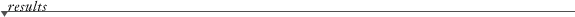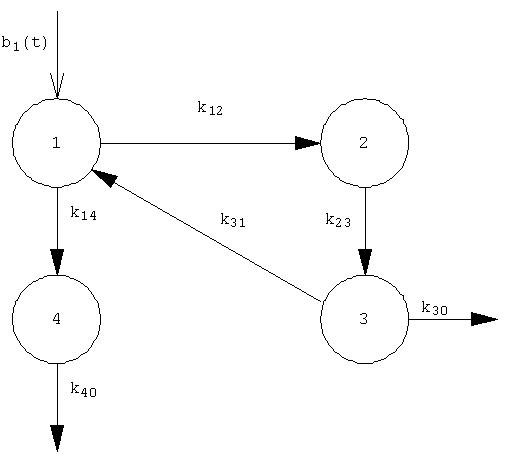Compartmental Modeling. Transfer Rate constants or function of the t
(Updated: 2021-12-12. Comments to: guillermo2046(at)gmail.com writing in the Subject:"BIOKMOD")

Here is applied SysModel package for solving linear compartmental model. The "compartmental matrix" shall be written: {{1,2,k12},...{i,j,kij},...,{n,n,knn}}, where kij is the transfer rate -clearance- from compartment i to compartment j, kij can be constant or function of t. The radioactive decay constant,if it available , will be written in the same units that the rate transfer constans. All units mush be coherent with kij, so if kij is in days^-1 the time t to be evaluated the retencion must be in days. In the case of that you are interested in the acumulate disintegrations kij must be in days^(-1) .

 Enter the compartmental matrix: {{1, 2, 0.83*(1 + 0.15*Cos[3 t])}, {1, 4, 1.94*(1+0.12*Sin[2 t])}, {2, 3, 0.008664}, {3, 0, 0.01155}, {3, 1, 0.0462}, {4, 0, 12}} Number of compartments:    Decay constant:   Initial conditions at time t = 0: Input function in each compartment : All values of this field must be {0,.., 0} if it is an impulsive single-input, because the inputs are the initial conditions. Time t to evaluate the content in each compartment (i.e.:{5, 20, 30}) : Range of t to be plotted:   From t-min   to t-max Time t, in days (kij in days^(-1)), to compute the acumulated disintegrations in each compartment :Differential equationSolutionPlot

Us (disintegrations in each compartment during a time t)The default values represents the iodine biokinetic model -see the figure - where compartment 1 is the blood, compartment 2 is the thyroid , compartment 3 is the rest of the body, compartment 4 is the blader, and "0" mean "out of the system" . The rate transfer values (clearance), in days^-1, is based in ICRP 78, however, for tutorial purposed, some of them are function of the time: k14 = 1.94*(1+0.12*Sin[2 t]), k12= 0.83*(1 + 0.15*Cos[3 t]), k23 = Log/80, k30 = 0.01155 and k31= 0.0462, and k40 = 12. Them the compartmental matrix is defined: {{1, 2, 0.83*(1 + 0.15*Cos[3 t])}, {1, 4, 1.94*(1+0.12*Sin[2 t]), {2, 3, 0.008664}, {3, 0, 0.01155}, {3, 1, 0.0462}, {4, 0, 12}}. Also it assumed the radioactive decay constant of I-131 that is Log/8.02 (days^-1). It assumed a continuouos input "1.2 Exp[-0.2 t]" in compartment 1, then the input is written: {0.1 Exp[-0.3 t], 0, 0, 0}. Also it is supossed as initial contition {1, 0, 0, 0}.